# 2018-ICLR-Variational image compression with a scale Hyper-prior

## 2. 引言

$R=\mathbb{E}_{\hat{\boldsymbol{y}} \sim m}\left[-\log _{2} p_{\hat{\boldsymbol{y}}}(\hat{\boldsymbol{y}})\right] \tag{1}$

## 3. 变分模型

$\mathbb{E}_{\boldsymbol{x} \sim p_{\boldsymbol{x}}} D_{\mathrm{KL}}\left[q \| p_{\tilde{\boldsymbol{y}} \mid \boldsymbol{x}}\right] = \mathbb{E}_{\boldsymbol{x} \sim p_{\boldsymbol{x}}} \mathbb{E}_{\tilde{\boldsymbol{y}} \sim q}[\underbrace{\cancel{\log q(\tilde{\boldsymbol{y}} \mid \boldsymbol{x})}}_{= 0} \underbrace{-\log p_{\boldsymbol{x} \mid \tilde{\boldsymbol{y}}}(\boldsymbol{x} \mid \tilde{\boldsymbol{y}})}_{\text {weighted distortion }} \underbrace{-\log p_{\tilde{\boldsymbol{y}}}(\tilde{\boldsymbol{y}})}_{\text {rate }}]+\text { const. } \tag{2}$

$p_{\tilde{\boldsymbol{y}} \mid \psi}(\tilde{\boldsymbol{y}} \mid \boldsymbol{\psi})=\prod_{i}\left(p_{y_{i} \mid \boldsymbol{\psi}^{(i)}}\left(\boldsymbol{\psi}^{(i)}\right) * \mathcal{U}\left(-\frac{1}{2}, \frac{1}{2}\right)\right)\left(\tilde{y}_{i}\right) \tag{3}$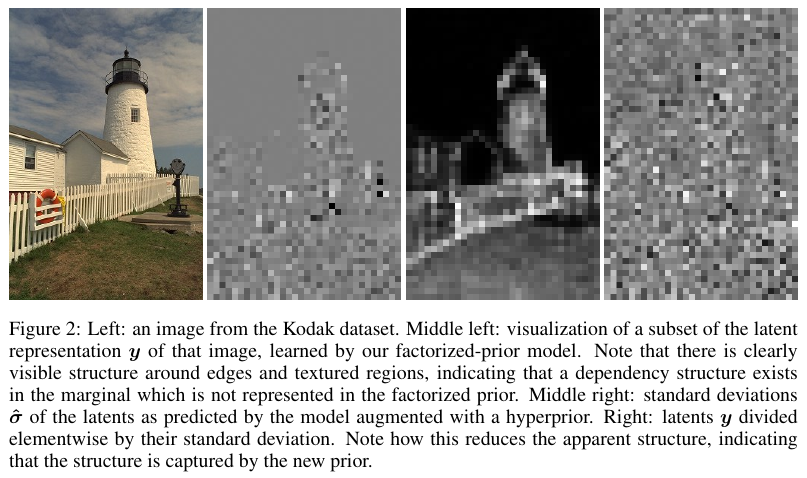## 4. 超先验引入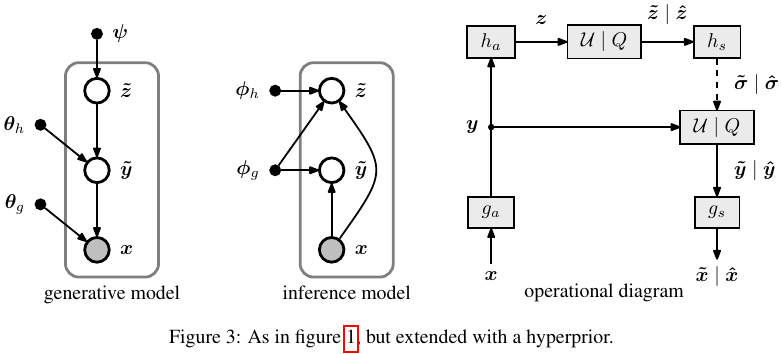\begin{align*} p_{\tilde{\boldsymbol{y}} \mid \tilde{\boldsymbol{z}}}\left(\tilde{\boldsymbol{y}} \mid \tilde{\boldsymbol{z}}, \boldsymbol{\theta}_{h}\right)=& \prod_{i}\left(\mathcal{N}\left(0, \tilde{\sigma}_{i}^{2}\right) * \mathcal{U}\left(-\frac{1}{2}, \frac{1}{2}\right)\right)\left(\tilde{y}_{i}\right) \\ & \text { with } \tilde{\boldsymbol{\sigma}}=h_{s}\left(\tilde{\boldsymbol{z}} ; \boldsymbol{\theta}_{h}\right) \tag{4} \end{align*}

\begin{align*} q\left(\tilde{\boldsymbol{y}}, \tilde{\boldsymbol{z}} \mid \boldsymbol{x}, \boldsymbol{\phi}_{g}, \boldsymbol{\phi}_{h}\right)=& \prod_{i} \mathcal{U}\left(\tilde{y}_{i} \mid y_{i}-\frac{1}{2}, y_{i}+\frac{1}{2}\right) \cdot \prod_{j} \mathcal{U}\left(\tilde{z}_{j} \mid z_{j}-\frac{1}{2}, z_{j}+\frac{1}{2}\right) \\ & \text { with } \boldsymbol{y}=g_{a}\left(\boldsymbol{x} ; \boldsymbol{\phi}_{g}\right), \boldsymbol{z}=h_{a}\left(\boldsymbol{y} ; \boldsymbol{\phi}_{h}\right) \end{align*} \tag{5}

$p_{\tilde{\boldsymbol{z}} \mid \psi}(\tilde{\boldsymbol{z}} \mid \boldsymbol{\psi})=\prod_{i}\left(p_{z_{i} \mid \psi^{(i)}}\left(\boldsymbol{\psi}^{(i)}\right) * \mathcal{U}\left(-\frac{1}{2}, \frac{1}{2}\right)\right)\left(\tilde{z}_{i}\right) \tag{6}$

\begin{align*} \mathbb{E}_{\boldsymbol{x} \sim p_{\boldsymbol{x}}} D_{\mathrm{KL}}\left[q \| p_{\tilde{\boldsymbol{y}}, \tilde{\boldsymbol{z}} \mid \boldsymbol{x}}\right] =&\ \mathbb{E}_{\boldsymbol{x} \sim p_{\boldsymbol{x}}} \mathbb{E}_{\tilde{\boldsymbol{y}}, \tilde{\boldsymbol{z}} \sim q}[\underbrace{\cancel{\log q(\tilde{\boldsymbol{y}}, \tilde{\boldsymbol{z}} \mid \boldsymbol{x})}}_{= 0} - \log p_{\boldsymbol{x} \mid \tilde{\boldsymbol{y}}}(\boldsymbol{x} \mid \tilde{\boldsymbol{y}}) \\ &-\log p_{\tilde{\boldsymbol{y}} \mid \tilde{\boldsymbol{z}}}(\tilde{\boldsymbol{y}} \mid \tilde{\boldsymbol{z}})-\log p_{\tilde{\boldsymbol{z}}}(\tilde{\boldsymbol{z}})]+\text { const. } \tag{7} \end{align*}

## 5. 模型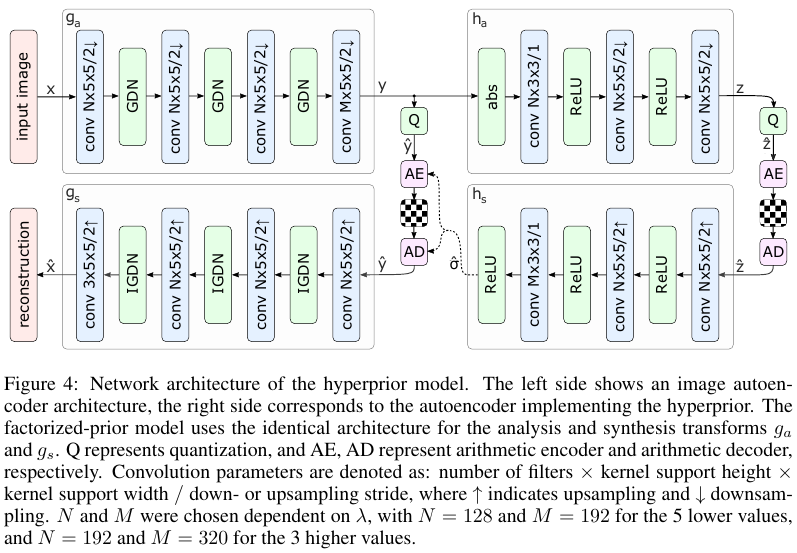## 6. 实验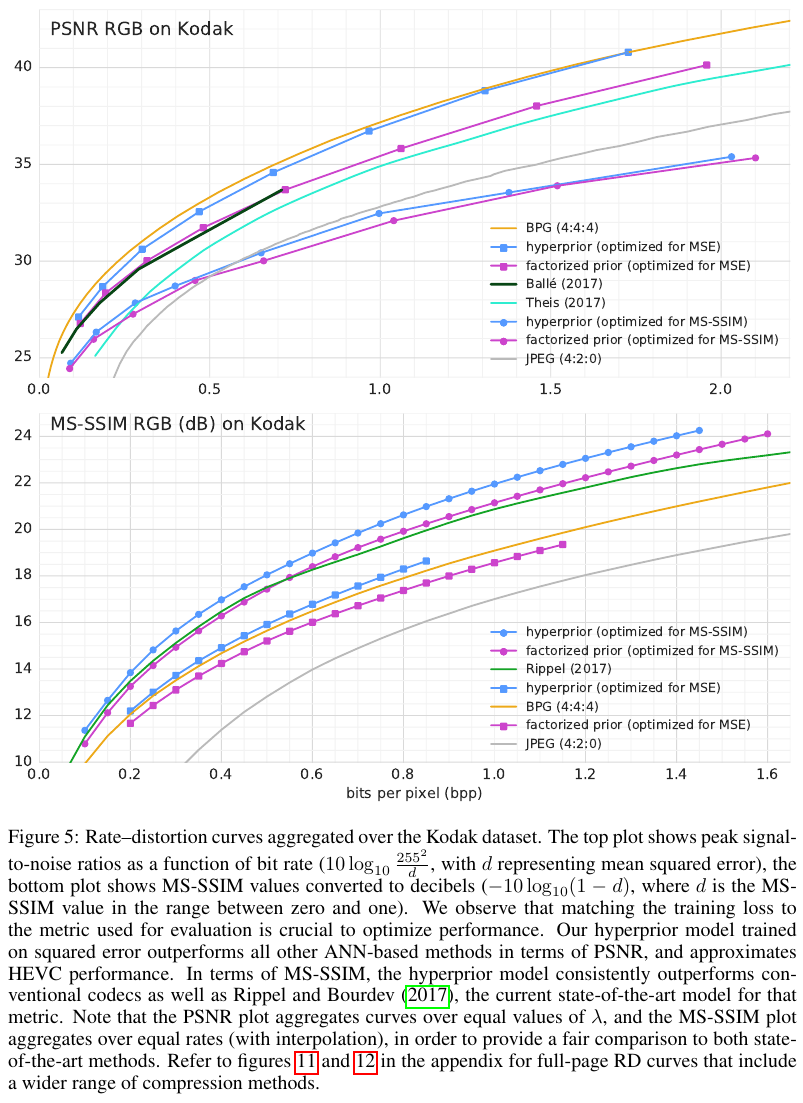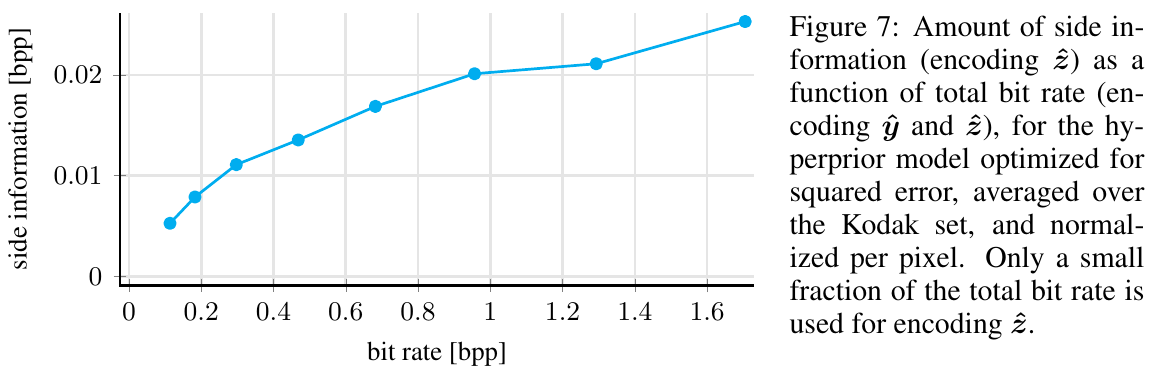## 附录

1. Ballé, J., Minnen, D., Singh, S., Hwang, S. J., & Johnston, N. (2018, February). Variational image compression with a scale hyperprior. In International Conference on Learning Representations.
2. Ballé, J., Laparra, V., & Simoncelli, E. P. (2017). End-to-end optimized image compression. In 5th International Conference on Learning Representations, ICLR 2017.